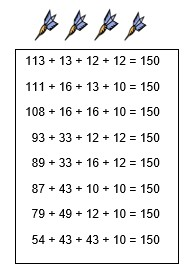Select Page

Each month, a new set of puzzles will be posted.  Come back next month for the solutions and a new set of puzzles, or subscribe to have them sent directly to you.

## Geometry Challenge

How is your Geometry? Can you find the measure of each missing angle (a – q) in the diagram below?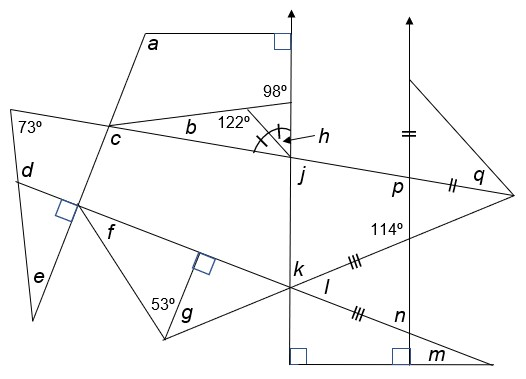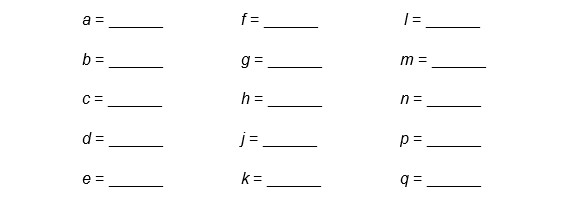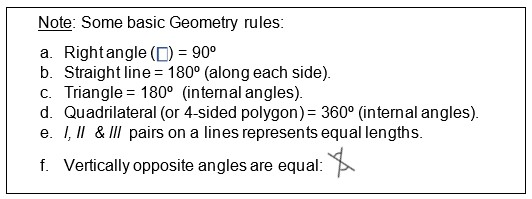## Feedback

There are more than one way of doing these puzzles and may well be more than one answer.  Please let me and others know what alternatives you find by commenting below.  We also welcome general comments on the subject and any feedback you'd like to give.

If you have a question that needs a response from me or you would like to contact me privately, please use the contact form.

## Get more puzzles!

If you've enjoyed doing the puzzles, consider ordering the books;

• Book One - 150+ of the best puzzles
• Book Two - 200+ with new originals and more of your favourites

Both in a handy pocket sized format.   Click here for full details.

## MIND-Xpander Numeric Puzzles

1. If QC = 14, TJ=10 & OK = 4, what would HA = ?
Solution: The numeric order of the 1st letter in the alphabet (where H=8) minus that of the 2nd letter (where A=1) gives value of 7.
2. What do you get if you divide 40 by a half and add 20 to the result?
Solution: (40 ÷ 1/2) + 20 = (40 x 2/1) + 20 = 100
3. How can you mathematically combine six 8’s to make 1000?
Solution: (888 – 88) ÷ .8 = 1000

## Safety-code Logic Puzzle

To open this safe you must press all the buttons in the correct order. The number on each button tells you how many squares to move, and the letters tells the direction (U = up, D = down, L = left and R = right). Which is the first button you must press to open this safe?

Solution: Start at ‘1R’ button in 3rd column, 2nd row.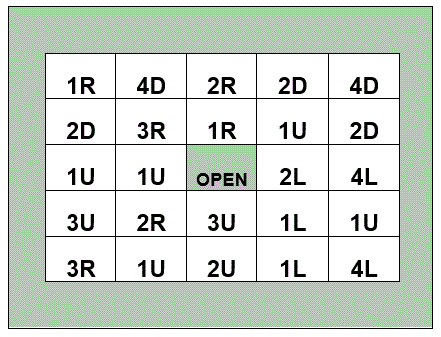## DARTS Numeric Puzzle

Using 4 darts and the following square dart board, find all combinations to score exactly 150. Numbers/boxes can be used more than once. All darts must be used, and each dart must be within one of the numbered boxes. (Note: there are several correct solutions.)